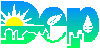Water/Wastewater Math Calculator
Loadings Calculator

Formula:

(F x C) x 8.34

=Lbs Loading

This calculator will calculate pounds of loading.

Please enter the data for flow and concentration.

 Enter Your Flow (millions of gallons per day) (example 2000 gallons per day = .002 MGD) MGD. Enter Concentration of Parameter, mg/l or PPM (mg/l of BOD, TSS, etc..) mg/l

 Click on this button to calculate pounds of loading
 Water/Wastewater math calculator. Select the calculator you want, then press GO! Select your Calculator - then press GO! Temperature Calculator ( C to F) Tank (or clarifier) Volume Calculator Removal Efficiency (wastewater) F/M (wastewater) Ratio Calculator